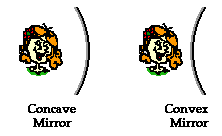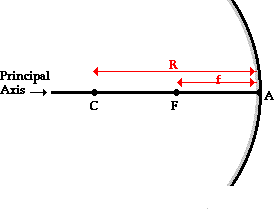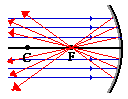Reflection and the Ray Model of Light - Lesson 3 - Concave Mirrors

# The Anatomy of a Curved Mirror

Thus far in this unit, our focus has been the reflection of light off flat surfaces and the formation of images by plane mirrors. In Lessons 3 and 4 we will turn our attention to the topic of curvedmirrors, and specifically curved mirrors that have a spherical shape. Such mirrors are called spherical mirrors. The two types of spherical mirrors are shown in the diagram on the right. Spherical mirrors can be thought of as a portion of a sphere that was sliced away and then silvered on one of the sides to form a reflecting surface. Concave mirrors were silvered on the inside of the sphere and convex mirrors were silvered on the outside of the sphere. In Lesson 3 we will focus on concave mirrors and in Lesson 4 we will focus on convex mirrors.

Beginning a study of spherical mirrors demands that you first become acquainted with some terminology that will be periodically used. The internalized understanding of the following terms will be essential during Lessons 3 and 4.

 Principal axis Center of Curvature Vertex Focal Point Radius of Curvature Focal Length

If a concave mirror were thought of as being a slice of a sphere, then there would be a line passing through the center of the sphere and attaching to the mirror in the exact center of the mirror. This line is known as the principal axis. The point in the center of the sphere from which the mirror was sliced is known as the center of curvature and is denoted by the letter C in the diagram below. The point on the mirror's surface where the principal axis meets the mirror is known as the vertex and is denoted by the letter A in the diagram below. The vertex is the geometric center of the mirror. Midway between the vertex and the center of curvature is a point known as the focal point; the focal point is denoted by the letter F in the diagram below. The distance from the vertex to the center of curvature is known as the radius of curvature (represented by R). The radius of curvature is the radius of the sphere from which the mirror was cut. Finally, the distance from the mirror to the focal point is known as the focal length (represented by f). Since the focal point is the midpoint of the line segment adjoining the vertex and the center of curvature, the focal length would be one-half the radius of curvature.The focal point is the point in space at which light incident towards the mirror and traveling parallel to the principal axis will meet after reflection.The diagram at the right depicts this principle. In fact, if some light from the sun were collected by a concave mirror, then it would converge at the focal point. Because the sun is such a large distance from the Earth, any light rays from the sun that strike the mirror will essentially be traveling parallel to the principal axis. As such, this light should reflect and pass through the focal point. A common Physics demonstration involves using a large demonstration mirror to set a pencil aflame in a matter of seconds. In the demonstration, the pencil is placed at the focal point and the concave mirror is pointed upwards towards the sun. Whatever rays of light from the sun that hit the mirror are focused at the point where the pencil is located. To the surprise of many, the heat is sufficient to ignite the pencil. Wow!

### Watch It!

A large concave mirror is used to focus light from the sun upon a pencil.

As we proceed through Lesson 3, we will observe the images formed by concave mirrors. Depending on the object location, the image could be enlarged or reduced in size or even the same size as the object; the image could be inverted or upright; and the image will be located in a specific region along the principal axis. To understand these relationships between object and image, you may need to review the vocabulary terms described on this page.

### We Would Like to Suggest ...Why just read about it and when you could be interacting with it? Interact - that's exactly what you do when you use one of The Physics Classroom's Interactives. We would like to suggest that you combine the reading of this page with the use of our Optics Bench Interactive or our Name That Image Interactive. You can find this in the Physics Interactives section of our website. The Optics Bench Interactive provides the learner an interactive enivronment for exploring the formation of images by lenses and mirrors. The Name That Image Interactive provides learners with an intensive mental workout in recognizing the image characteristics for any given object location in front of a curved mirror.

### Check Your Understanding

1. The surface of a concave mirror is pointed towards the sun. Light from the sun hits the mirror and converges to a point. How far is this converging point from the mirror's surface if the radius of curvature (R) of the mirror is 150 cm?

2. It's the early stages of the Concave Mirror Lab. Your teacher hands your lab group a concave mirror and asks you to find the focal point. What procedure would you use to do this?

Next Section: